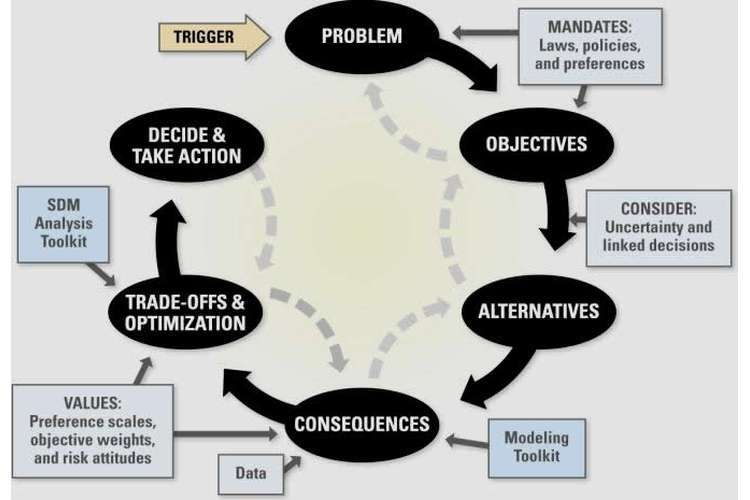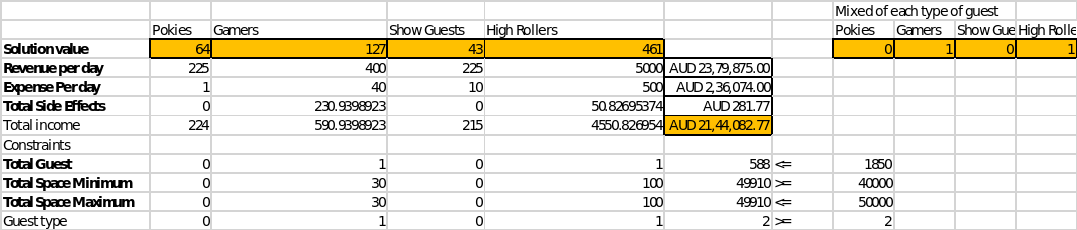# Data Analysis and Decision Modelling Oz Assignments## Introduction:

In this assignment the main objective is to maximize the revenue of the casino recently owned by Holiday Entertainment Corporation (HEC) by choosing a specific strategy. Hence, a total of 3 strategies are evaluated by the method of optimization by using Linear Programming in excel solver and then the best business strategy is chosen. The three models that are evaluated are base model, alternative 1 and alternative 2 model. In all the models the optimum number of pokies, gamers, show guests and high rollers that will generate the maximum income are calculated with some constraints as specified below.

### Base model:

In this model the base revenue, side effects, expenses, minimum square feet needed, minimum and maximum of each guest is given and the Linear Programming model is formed in excel as given below.

 Pokies(P) Gamers(G) Show guests(S) High Rollers(H) Base revenue 200 300 100 5000 Food and drink revenue 25 100 125 0 Side effects 0 more (H/10)*2 less (P/25)*5 less (P/25)*2 less (P/25)*5 more (G/25)*10 Expenses -1 -40 -10 -500 Square feet needed 15 30 10 100 Total revenue 225 400 225 5000 Expenses -1 -40 -10 -500 Income 224 360 215 4500 Objective Function 224*P + 360*G + 215*S + 4500*H + H/5 - P/5 - 2*P/25 - P/5 + 2*G/5 MAXIMIZE 5588*P/25 + 1802*G/5 + 215*S + 22501*H/5 Constraints P*15 + G*30 + S*10 + H*100 <= (50000- 5000 -5000) P + G + S + H <= 1850 Additional constraints 600 <= P <= 800 400 <= G <= 800 300 <= S <= 800 20 <= H <= 60

The optimum number of guests satisfying all the constraints are

Pokies = 600, Guests = 655, Show guests = 535 and High rollers = 60

And the maximized revenue is \$755211.

Alternative 1 model:

In this model the conditions of base model is considered along with the condition that the buffet dining area can be reduced from 5000 square feet to 1500 square feet and the maximum number of gamers and high rollers can be removed such that the revenue generated is not less than the same in base model. The LP which is formulated in excel solver is given below.

 Pokies(P) Gamers(G) Show guests(S) High Rollers(H) Base revenue 200 300 100 5000 Food and drink revenue 25 100 125 0 Side effects 0 more (H/10)*2 less (P/25)*5 less (P/25)*2 less (P/25)*5 more (G/25)*10 Expenses -1 -40 -10 -500 Square feet needed 15 30 10 100 Total revenue 225 400 225 5000 Expenses -1 -40 -10 -500 Income 224 360 215 4500 Objective Function MINIMIZE G+H Constraints P*15 + G*30 + S*10 + H*100 <= (50000- 1500 -5000) P + G + S + H <= 1850 5588*P/25 + 1802*G/5 + 215*S + 22501*H/5 >= 755211 Additional constraints 600 <= P <= 800 400 <= G <= 800 300 <= S <= 800 20 <= H <= 60

Now, solving the model, the total income generated in this model is 755315.60 and the optimal number of guests are Pokies = 800, Gamers = 644, Show guests = 346 and High rollers = 60.

In this model the utilized square feet by the guests is 40780 square feet. Now, as per the condition the minimum number of gamers and high rollers to meet the minimum income is 704.

### Alternative 2 model:

In this model the mixed number of guests required (by considering at least two type of guests in the model) to generate the maximum income and both buffet dining area and show dining area can be compromised for more space. Also, the minimum income from this model should be at least \$1500000 with the minimum and maximum of each type of guests can be modified. The LP of this problem as formed in excel is given below. All the other constraints in the base model is kept in this model also.Now, the mixed type of guests is considered as a binary variable i.e. in the solution if a particular type of guest is 0, then in the optimized revenue that type of guest is not considered. From the solution it is evident that the Gamers and High rollers is 1, that means only 127 gamers and 461 high rollers will maximize the revenue satisfying all the constraints.

The maximized revenue of this model is \$2144082 and the total area that is utilized is 49910 by a total of 588 guests (127 gamers and 461 high rollers).

#### Conclusion:

Now, comparing the three models above namely base model, alternative 1 model and the alternative 2 model it is seen that the income is maximum in the alternative 2 model and hence the best strategy for maximizing the income from the casino is to employ the alternative 2 model by Holiday Entertainment Corporation Law.